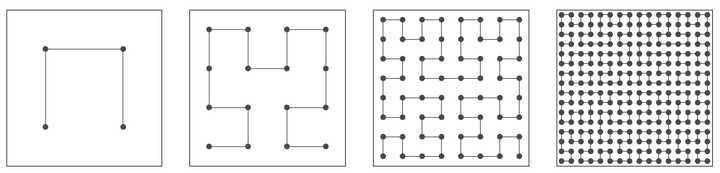# Extensible Grids -- Uniform Sampling on a Space-Filling Curve### 摘要

We study the properties of points in $[0,1]^d$ generated by applying Hilbert’s space-filling curve to uniformly distributed points in $[0,1]$. For deterministic sampling we obtain a discrepancy of $O(n^{-1/d})$ for $d\ge2$. For random stratified sampling, and scrambled van der Corput points, we get a mean squared error of $O(n^{-1-2/d})$ for integration of Lipschitz continuous integrands, when $d\ge3$. These rates are the same as one gets by sampling on $d$ dimensional grids and they show a deterioration with increasing $d$. The rate for Lipschitz functions is however best possible at that level of smoothness and is better than plain IID sampling. Unlike grids, space-filling curve sampling provides points at any desired sample size, and the van der Corput version is extensible in $n$. We also introduce a class of piecewise Lipschitz functions whose discontinuities are in rectifiable sets described via Minkowski content. Although these functions may have infinite variation in the sense of Hardy and Krause, they can be integrated with a mean squared error of $O(n^{-1-1/d})$. It was previously known only that the rate was $o(n^{-1})$. Other space-filling curves, such as those due to Sierpinski and Peano, also attain these rates, while upper bounds for the Lebesgue curve are somewhat worse, as if the dimension were $\log_2(3)$ times as high.

Journal of the Royal Statistical Society: Series B, 78(4), 917-931